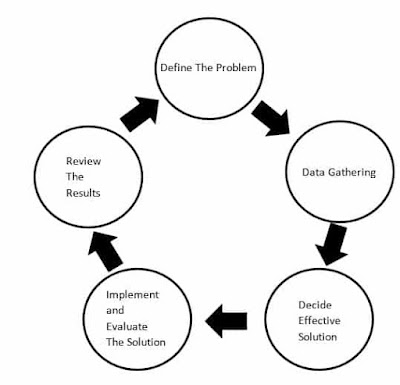# Problem Solving Techniques | Definition Steps Process Techniques EasilyProblem Solving Techniques | Definition Steps Process Techniques Easily

## Problem Solving Techniques

The computer is a multi-purpose electronic machine that is used for storing, organizing, and processing the data by using a set of programs.
The computer is not able to find the solution to a problem rather a computer is a machine which follows the instructions given by the programmer to solve any problem. So all the efforts needed to understand the problem and define the procedure to solve that problem is done by a programmer (a human being).
First thing in problem-solving is to understand the problem properly and then define proper steps to
produce a perfect solution for that problem.

### Meaning Of Problem

Before a program is written for solving a problem, it is important to define the problem clearly.
Problem is a situation or issue or condition which needs to solve to achieve the goal. For most software projects the system-analysts approach system users to collect user requirements and define the problem that the system aims to solve.
System-analysts typically look at the following issues:
• What the problem is?
• What input is required for achieving the expected output?
• The expected output of the problem.
• The current method of solving the problem.
• Can the problem or part of the problem be more effectively solved by a software solution?
what is problem solving ?
what is problem solving skills?

### Steps In Problem SolvingSteps In Problem Solving

how to improve problem solving skills
how to develop problem solving skills
problem solving skills

### Problem-solving techniques

Before we talk about the techniques , you should know the algorithm and flowchart.

The algorithm is a set of ordered instructions that are written in simple English Language.
The algorithm defines the step by step logic for a program to solve specific problem.
In this type of problem-solving the required inputs and expected outputs are identified and according to that the processing will be done.

A flowchart is a good problem-solving technique. The flowchart is a graphical representation of an algorithm. The sequence of steps in the algorithm is maintained and represented in the flowchart by using some standard symbols such as rectangles and directed lines.

#### Some Problem-solving Techniques List

1. Trial and error technique
2. Brainstorming technique
3. Divide and conquer technique
4. Solve by analogy
5. The building block approach
6. Merging solution
Trial and error technique
Trial and error is also known as Generate and test. It is a way of solving problems through repeated attempts, trying variant data on the sequence of steps until you are successful

Brainstorming technique
It is a group activity. Group members are gathering together to discuss a problem and give solutions to it.

Divide and conquer technique
This technique is useful when the problem is big and can take a longer time to identify and solve.
This technique states that the problem is divided into small sub-problems, again these sub-problems are subdivided into sub-sub-problems, and so on, until each part of the problem becomes easier to solve.
After solving the smallest sub-problem all the results get conquer to meet the actual complete solution to the original problem.

Solve by analogy
The idea behind this technique is that it looks for an existing solution for a similar type of problem and apply it to the given problem.

The building block approach
It combines the idea of solving by analogy and divide and conquer techniques. This technique checks if any solutions for smaller pieces of the problem exist.

Merging solution
The idea behind this is to combine existing solutions and merge them on a step by step basis.

#### Conclusion: We have covered what is problem solving,,problem solving steps,solve a question of problem solving techniques and decision making skills

Sharing Is Caring